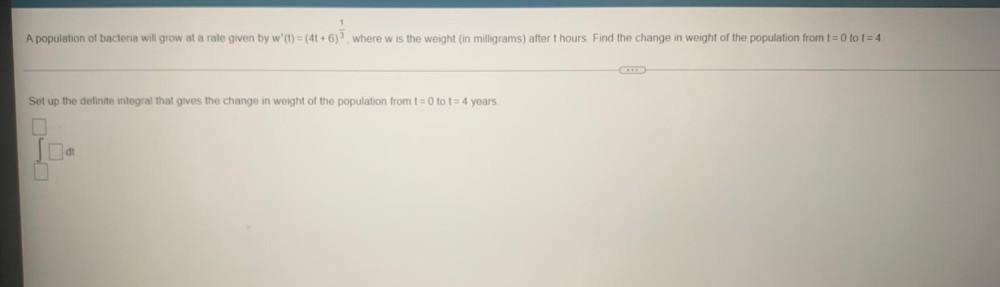Question:

# 1 A population of bacteria will grow at a rate given by w' (t) = (4 + 6), where w is the weight (in milligrams) after t hours Fi1 A population of bacteria will grow at a rate given by w' (t) = (4 + 6), where w is the weight (in milligrams) after t hours Find the change in weight of the population from t=0 to t=4 Set up the definite integral that gives the change in weight of the population from t=0 to t = 4 years dt